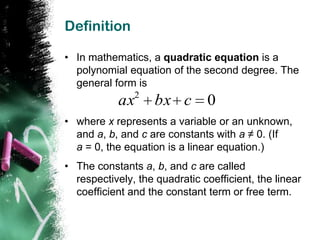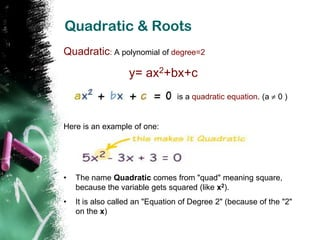Successfully reported this slideshow.

215

Share×
1 of 16
1 of 16

215

Share

## More Related Content

### Related Books

Free with a 14 day trial from Scribd

See all

### Related Audiobooks

Free with a 14 day trial from Scribd

See all

1. 1. Quadratic Equations By: Sushmita Kumari Roll: 03 Class: X ‘B’
2. 2. Definition • In mathematics, a quadratic equation is a polynomial equation of the second degree. The general form is 2 ax bx c 0 • where x represents a variable or an unknown, and a, b, and c are constants with a ≠ 0. (If a = 0, the equation is a linear equation.) • The constants a, b, and c are called respectively, the quadratic coefficient, the linear coefficient and the constant term or free term.
3. 3. Quadratic & Roots Quadratic: A polynomial of degree=2 y= ax2+bx+c is a quadratic equation. (a 0) Here is an example of one: • The name Quadratic comes from "quad" meaning square, because the variable gets squared (like x2). • It is also called an "Equation of Degree 2" (because of the "2" on the x)
4. 4. Roots  A real number α is called a root of the quadratic equation ,a≠0 if aα2 + bα2 + c = 0.  If α is a root of ,then we say that: (i) x= α satisfies the equation ax2+bx+c =0 Or (ii) x= α is a solution of the equation ax2+bx+c =0  The Root of a quadratic equation ax2+bx+c =0 are called zeros of the polynomial ax2+bx+c .
5. 5. More Examples of Quadratic Equations  In this one a=2, b=5 and c=3.  This one is a little more tricky: Where is a? In fact a=1, as we don't usually write "1x2“ b = -3 and where is c? Well, c=0, so is not shown.  Oops! This one is not a quadratic equation, because it is missing x2 (in other words a=0, and that means it can't be quadratic)
6. 6. Hidden Quadratic Equations! So far we have seen the "Standard Form" of a Quadratic Equation: But sometimes a quadratic equation doesn't look like that..! Here are some examples of different form: In disguise In Standard Form a, b and c x2 = 3x -1 Move all terms to x2 - 3x + 1 = 0 a=1, b=-3, c=1 left hand side 2(w2 - 2w) = 5 Expand (undo the 2w2 - 4w - 5 = 0 a=2, b=-4, c=-5 brackets), and move 5 to left z(z-1) = 3 Expand, and move a=1, b=-1, c=-3 3 to left z2 - z - 3 = 0 5 + 1/x - 1/x2 = 0 Multiply by x2 5x2 + x - 1 = 0 a=5, b=1, c=-1
7. 7. How To Solve It? There are 3 ways to find the solutions:  We can Factor the Quadratic (find what to multiply to make the Quadratic Equation)  We can Complete the Square, or  We can use the special Quadratic Formula: Thus ax2+bx+c =0 has two roots α and β, given by b b 2 4ac b b 2 4 ac α= β= 2a 2a
8. 8. Discriminant  The expression b2 - 4ac in the formula  It is called the Discriminant, because it can "discriminate" between the possible types of answer.It can be denoted by “D”  when b2 - 4ac, D is positive, you get two real solutions  when it is zero you get just ONE real solution (both answers are the same)  when it is negative you get two Complex solutions Value of D Nature of Roots Roots D>0 Real and Unequal [(-b±√D)/2a] D=0 Real and Equal Each root = (-b/2a) D<0 No real roots None
9. 9. Using the Quadratic Formula Just put the values of a, b and c into the Quadratic Formula, and do the calculation Example: Solve 5x² + 6x + 1 = 0 Coefficients are: a = 5, b = 6, c = 1 Quadratic Formula: x = [ -b ± √(b2-4ac) ] / 2a Put in a, b and c: 6 62 4 5 1 x= 2 5 6 36 20 Solve: x = 10 6 16 x= 10 6 4 x= 10 x = -0.2 or -1
10. 10. Continue..  Answer: x = -0.2 or x = -1  Check -0.2: 5×(-0.2)² + 6×(-0.2) + 1 = 5×(0.04) + 6×(-0.2) + 1 = 0.2 -1.2 + 1 =0  Check -1: 5×(-1)² + 6×(-1) + 1 = 5×(1) + 6×(-1) + 1 =5-6+1 =0
11. 11. Factoring Quadratics  To "Factor" (or "Factorize") a Quadratic is to find what to multiply to get the Quadratic It is called "Factoring" because you find the factors (a factor is something you multiply by)  Example The factors of x2 + 3x - 4 are: (x+4) and (x-1) Why? Well, let us multiply them to see: (x+4)(x-1) = x(x-1) + 4(x-1) = x2 - x + 4x - 4 = x2 + 3x – 4 • Multiplying (x+4)(x-1) together is called Expanding. • In fact, Expanding and Factoring are opposites:
12. 12. Examples of Factor To solve by factoring: 1. Set the equation equal to zero. 2. Factor. The factors will be linear expressions. 3. Set each linear factor equal to zero. 4. Solve both linear equations. Example: Solve by factoring x2 + 3x = 0 x2 + 3x = 0 set equation to zero x( x + 3) = 0 factor x=0 , x+3=0 x = -3 set the linear factors equal to zero and solve the linear equation
13. 13. Completing the Square Solving General Quadratic Equations by Completing the Square: "Completing the Square" is where we take a Quadratic Equation : ax2 + bx + c = 0 and turn into a(x+d)2 + e = 0 We can use that idea to solve a Quadratic Equation (find where it is equal to zero). But a general Quadratic Equation can have a coefficient of a in front of x2: But that is easy to deal with ... just divide the whole equation by "a" first, then carry on.
14. 14. Steps Now we can solve Quadratic Equations in 5 steps:  Step 1 Divide all terms by a (the coefficient of x2).  Step 2 Move the number term (c/a) to the right side of the equation.  Step 3 Complete the square on the left side of the equation and balance this by adding the same value to the right side of the equation.  Step 4 Take the square root on both sides of the equation.  Step 5 Add or subtract the number that remains on the left side of the equation to find x.
15. 15. Example Example 1: Solve x2 + 4x + 1 = 0 Step 1 can be skipped in this example since the coefficient of x2 is 1 Step 2 Move the number term to the right side of the equation: x2 + 4x = -1 Step 3 Complete the square on the left side of the equation and balance this by adding the same number to the right side of the equation: x2 + 4x + 4 = -1 + 4 (x + 2)2 = 3 Step 4 Take the square root on both sides of the equation: x + 2 = ±√3 = ±1.73 (to 2 decimals) Step 5 Subtract 2 from both sides: x = ±1.73 – 2 = -3.73 or -0.27
16. 16. BIBLIOGRAPHY  Internet (Wikipedia,www.mathsisfun.com)  Secondary School Mathematics (R.S. Aggarwal)